# 11-1100/Simplicity of the Alternating Group

The following is the proof for the simplicity of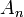$A_n$. It is available individually in pdf format, can be found in the course notes, or on another user page.

Theorem: The alternating group$A_n$ is simple for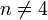$n\neq 4$.

Note that for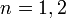$n=1,2$ this is trivial. For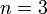$n=3$ we have that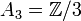$A_3 = \mathbb Z/3$ which is an abelian group of prime-order, and hence simple.

We know that for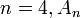$n=4, A_n$ is not simple. Indeed, we have seen that there is a non-trivial homomorphism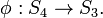$\phi:S_4 \to S_3.$ By restricting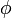$\phi$ to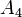$A_4$ we still have a non-trivial homomorphism whose kernel is non-trivial. This kernel is a normal subgroup.

We will need the following Lemmas for our proof.

Lemma: Every element of$A_n$ is a product of 3-cycles.

Proof

Every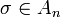$\sigma \in A_n$ is a product of an even number of 2-cycles. Without loss of generality, we will demonstrate this on the following "cycles. Indeed,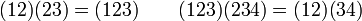$(12)(23) = (123) \qquad (123)(234) = (12)(34)$

Lemma: If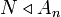$N \triangleleft A_n$ contains a 3-cycle, then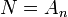$N=A_n$.

Proof

Without loss of generality, we can consider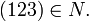$(123) \in N.$ We want to show that for all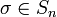$\sigma \in S_n$ we must have that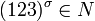$(123)^\sigma \in N$. If$\sigma \in A_n$ then this is clear since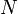$N$ is normal in$A_n$; otherwise, take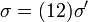$\sigma = (12)\sigma'$ with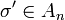$\sigma' \in A_n$. Since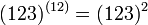$(123)^{(12)} = (123)^2$ we have that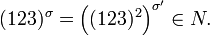$(123)^\sigma = \left((123)^2 \right)^{\sigma'} \in N.$ So$N$ contains all three cycles.

Proof [Proof of Theorem]

Let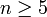$n \geq 5$. By the previous two lemmas, it is sufficient to show that$N$ contains a three cycle.
"Case 1:"$N$ contains an element with cycle length at least 4.
Let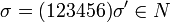$\sigma = (123456)\sigma' \in N$. Now$N$ is normal in$A_n$ so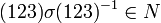$(123) \sigma (123)^{-1} \in N$. Similarly, multiplying by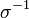$\sigma^{-1}$ will keep the element in$N$, so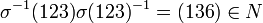$\sigma^{-1} (123) \sigma (123)^{-1} = (136) \in N$ and$N$ contains a three cycle.
Case 2:$N$ contains an element with two cycles of length 3.
Let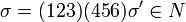$\sigma = (123)(456) \sigma' \in N$. Then by the same reasoning as before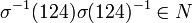$\sigma^{-1}(124)\sigma(124)^{-1} \in N$ and can be computed as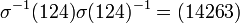$\sigma^{-1}(124)\sigma(124)^{-1} = (14263)$. We can now use Case 1 to deduce that$N$ has a three cycle.
Case 3:$N$ contains an element that is a three-cycle and a product of disjoint transpositions. Write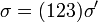$\sigma = (123)\sigma'$ and note that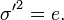${\sigma'}^2 = e.$
Then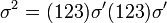$\sigma^2 = (123)\sigma'(123)\sigma'$ but the elements of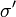$\sigma'$ are disjoint with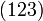$(123)$, and we conclude that$\sigma'$ and$(123)$ commute; that is,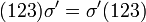$(123)\sigma' = \sigma'(123)$. Thus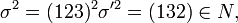$\sigma^2 = (123)^2 \sigma'^2 = (132) \in N,$ and$N$ contains a three cycle.
Case 4: Finally, consider the case when the element of$N$ is a product of disjoint 2-cycles.
Write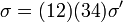$\sigma = (12)(34) \sigma'$. By previous rationale, we know that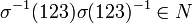$\sigma^{-1} (123) \sigma (123)^{-1} \in N$ and can be computed as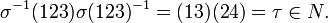$\sigma^{-1} (123) \sigma (123)^{-1} = (13)(24) = \tau \in N.$ We can view this as a sort of purification, in that we have simplified a product of disjoint 2-cycles of arbitrary length into the case of two disjoint 2-cycles. Applying this procedure again, we get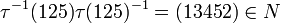$\tau^{-1}(125)\tau(125)^{-1} = (13452) \in N$ and we again refer to Case 1 to conclude$N$ contains a three cycle.

Note that this last case is the only case in which we did not assume "5" was part of the hypothesis, but needed to use it. Hence we need$n \geq 5$ for this case to hold.